## Chemistry Formulas for Atomic Structure

### Chemistry Formulas from Bohr’s Model of Atom

#### ·         Angular momentum of electron in nth orbit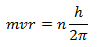Where, m = Mass of the electron,
v = velocity of electron,
r = radius of the orbit,
h = Planck’s constant,
n = no. of orbit in which electron is present,

#### ·         Energy of electron in nth orbit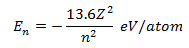Where, Z = Atomic No. of Electron,

#### ·         Energy absorbed or released in an electron jump (E)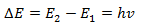#### ·         Radius of orbits of hydrogen like species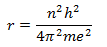For hydrogen atom Z = 1, for first orbit n = 1,
On substituting values of the constants
h = 6.62x10-27erg sec,
m = 9.1x10-28g,
e = 4.8x10-10
we get,
r = 0.529 Å
So, radius of first orbit of hydrogen atom is 0.529 Å.

rn = 0.529n2/Z Å

#### ·         Velocity of electron in nth orbit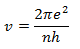On substituting values of the constants
We get,

#### ·         No. of revolution per second made by an electron around the nucleus of atom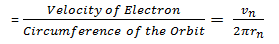#### ·         Energy of electron in nth orbit (En)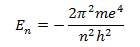On substituting values of the constants
We get,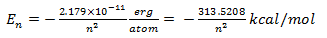In general,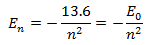#### ·         Energy of electron in a Hydrogen Atom in different energy levels

 Energy Level E (Joules/atom) E (eV/atom) E (kcal/mol) 1 -21.79x10-19 -13.6 -313.5 2 -5.42x10-19 -3.4 -78.4 3 -2.41x10-19 -1.51 -38.84 4 -1.36x10-19 -0.85 -19.6 5 -0.87x10-19 -0.544 -12.5 Infinite 0 0 0

#### ·         Frequency or wave length of emitted radiation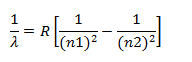Where, λ = wavelength of emitted radiations
R = Rydberg constant for Hydrogen atom

#### ·         Number of spectral lines produced when an electron drops from nth level to ground level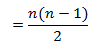### Chemistry Formulas from Photoelectric Effect

E = hv

#### ·         Total energy,

Total Energy = (mv2/2) + w
Where, w = energy required to remove the electron.

### Chemistry Formulas from Wave Mechanical Concept of Atom

#### ·         De Broglie’s Equation,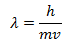Where, m = mass of particle,
v = velocity of the particle,
h = Planck’s Constant,
or,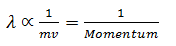### Chemistry Formulas from Heisenberg’s Uncertainty Principle

#### ·        Heisenberg’s Uncertainty Principle,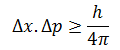Where,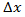= uncertainty in the position of the particle,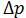= uncertainty in the momentum of the particle,
Also,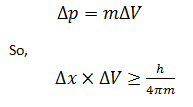### Chemistry Formulas from Quantum Numbers

#### ·         Principle Quantum Number (n),

Maximum no. of electrons in n principle quantum number = 2n2

#### ·         Azimuthal Quantum Number (l),

For the given value of principle quantum number (n), azimuthal quantum number (l), may have all integral values from 0 to (n-1)

#### ·         Magnetic Quantum Number (m),

No. of orbitals in a sub-shell = 2 l +1

#### ·         Spin Quantum Number (s),

For spinning of electron about its own axis